# Else If Statement CLearn C - C tutorial - Else if statement c - C examples - C programs

## Else If Statement C - Definition and Usage

• In C- Programming the Else If statement works like, the if condition works if the condition is true, if condition is false the else condition works, certainly further on if the else condition is false once again the else condition will be executed.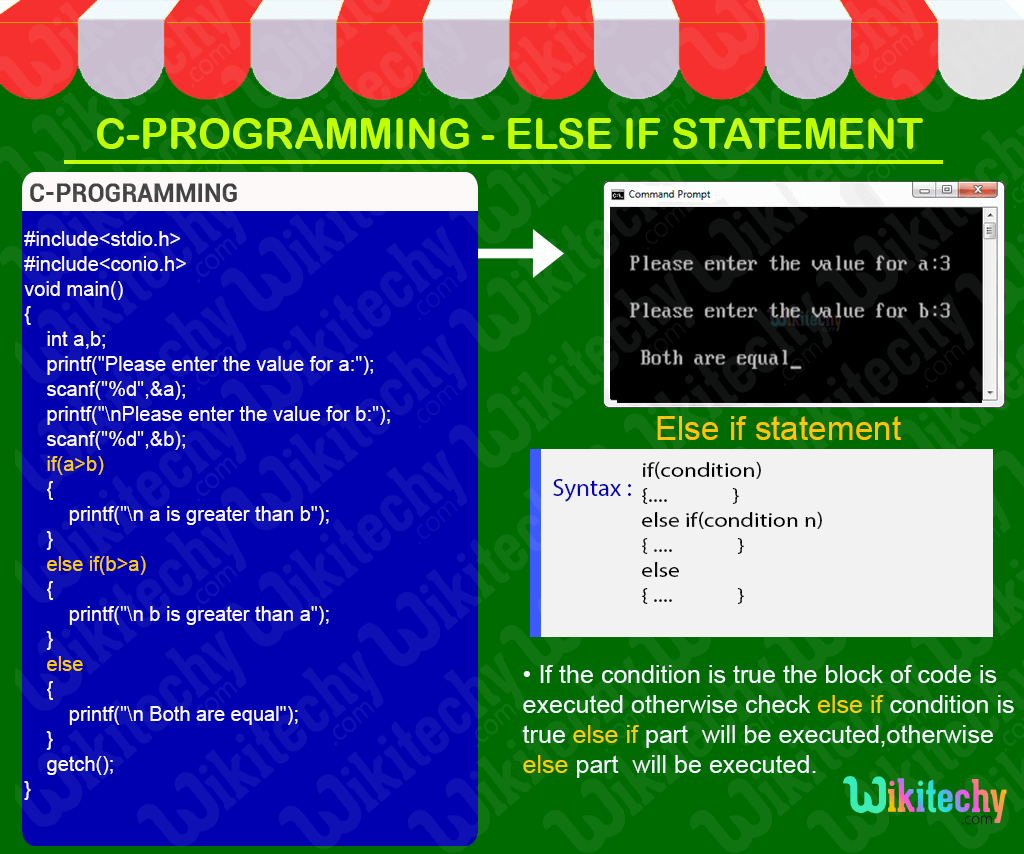## C Code - Explanation :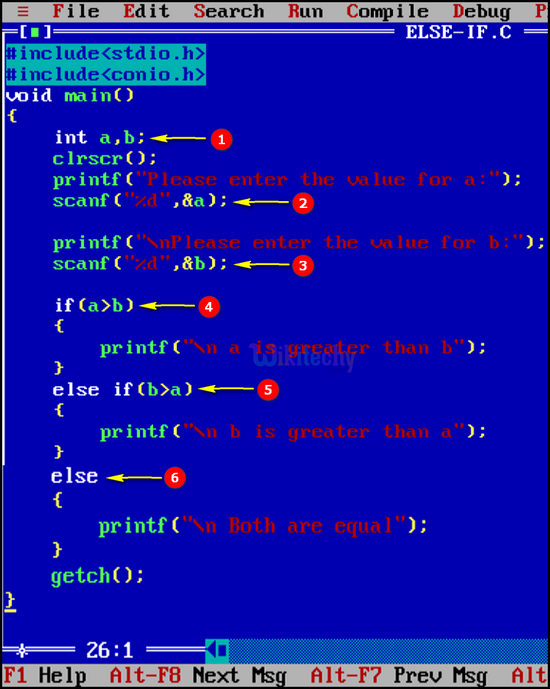1. Here we declare the variable “a” and “b” as integer.
2. In this statement we get the value of the variable “a” using scanf statement.
3. In this statement we get the value of the variable “b” using scanf statement.
4. In this statement we check the condition for the value “a” is greater than “b” which means, it prints “a is greater than b”.
5. In this statement we check the condition for the value “b” is greater than “a” which means, it prints “b is greater than a”.
6. If both the condition is false it prints “Both the values are equal”.

## Sample Output - Programming Examples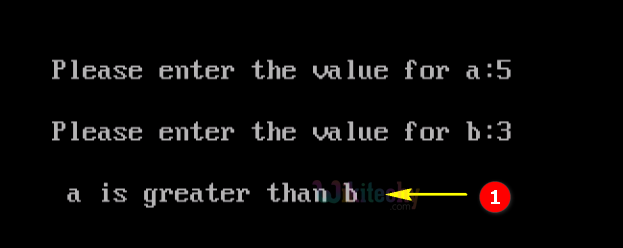1. Here in this output we get the “a” value as “5” and “b” value as “3” ,where the if condition section will be satisfied so the output will be displayed as “a is greater than b”.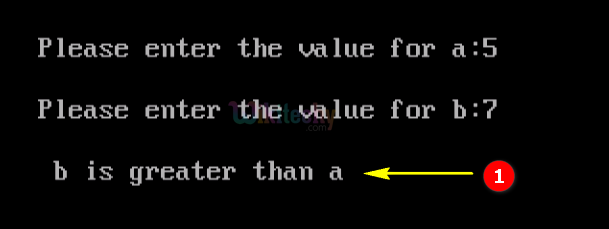1. Here in this output we get the “a” value as “5” and “b” value as “7”, where the else if condition section will be satisfied so the output will be displayed as “b is greater than a”.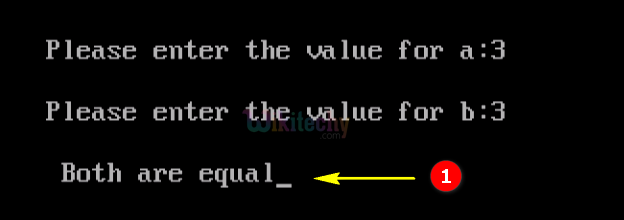1. Here in this output we get the, “a” value as “3” and “b” value as “3”, where the else condition section will be satisfied so the output will be displayed as “Both are equal”.